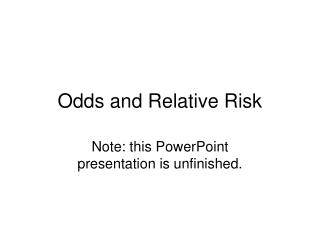DownloadDownload PresentationOdds and Relative Risk

# Odds and Relative Risk

Télécharger la présentation## Odds and Relative Risk

- - - - - - - - - - - - - - - - - - - - - - - - - - - E N D - - - - - - - - - - - - - - - - - - - - - - - - - - -
##### Presentation Transcript

1. Odds and Relative Risk Note: this PowerPoint presentation is unfinished.

2. Probabilities

3. Probabilities Suppose that in 2005-06 a particular 2-year college graduated 300 students from a cohort of 700 students. In this case the probability of graduating is:

4. Probabilities Suppose that in 2005-06 a particular 2-year college graduated 300 students from a cohort of 700 students. In this case the probability of graduating is:

5. Odds

6. Odds Hence the odds of graduating from the cohort are:

7. Odds Hence the odds of graduating from the cohort are:

8. Odds Hence the odds of graduating from the cohort are: i.e., the odds of graduating are about 3 to 4.

9. Conditional Probability The conditional probability of an event is the probability of an event given the occurrence of some other event. The symbol represents the intersection of G and A). In Table 1 (on the next slide) the probability of a parent attending college (A) is 325/700 = .464. The Conditional probability is given by

10. Table 1 Numbers of students graduating from college 2005-2006 cross-tabulated with whether or not their parents attended college

11. Table of Conditional Probabilities

12. Conditional odds are ratios of conditional probabilities.

13. Conditional odds are ratios of conditional probabilities.

14. Conditional Odds Conditional odds are ratios of conditional probabilities.

15. Odds Ratio (OR) The OR is the ratio of Odds for one group to the Odds for another group.

16. Odds Ratio (OR) The OR is the ratio of Odds for one group to the Odds for another group. The odds of graduating when parents have attended college are nearly 22 times greater than when parents have not attended college.

17. Risk The term risk is just another term for a conditional probability. For example, in the current example, the risk failing to graduate NG when no parent has attended college (NA) is the conditional probability of not graduating when no parent attended college, or Risk {NG|NA} = Pr{NG | NA} =.867.

18. Relative-risk (or Risk Ratio) (RR) The RR is the ratio of two conditional probabilities. The relative risk of failing to graduate given a parent has attended college Relatively, speaking, risk of not graduating when neither parent has attended college is almost four times greater than when a parent has attended college.